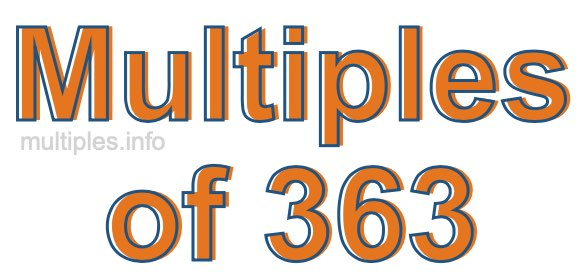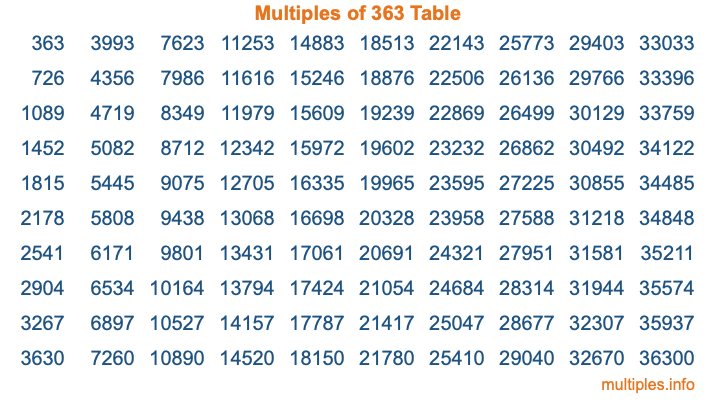Multiples of 363Welcome to the Multiples of 363 page. Here we will first teach you everything you will ever need to know about the multiples of 363, and then give you a study guide summary of everything we taught you to make sure you remember it all. Use this page to look up facts and learn information about the multiples of 363. This page will make you a multiples of three hundred sixty-three expert!

Definition of Multiples of 363
Multiples of 363 are all the numbers that when divided by 363 equal an integer. Each of the multiples of 363 are called a multiple. A multiple of 363 is created by multiplying 363 by an integer.

Therefore, to create a list of multiples of 363, you start with 1 multiplied by 363, then 2 multiplied by 363, then 3 multiplied by 363, and so on for as long as you want. Thus, the list of the first five multiples of 363 is 363, 726, 1089, 1452, and 1815. To see a larger list of multiples of 363, see the printable image of Multiples of 363 further down on this page. We also have a category where you can choose any nth multiple of 363.

Multiples of 363 Checker
The Multiples of 363 Checker below checks to see if any number of your choice is a multiple of 363. In other words, it checks to see if there is any number (integer) that when multiplied by 363 will equal your number. To do that, we divide your number by 363. If the the quotient is an integer, then your number is a multiple of 363.

Is  a multiple of 363?

Least Common Multiple of 363 and ...
A Least Common Multiple (LCM) is the lowest multiple that two or more numbers have in common. This is also called the smallest common multiple or lowest common multiple and is useful to know when you are adding our subtracting fractions. Enter one or more numbers below (363 is already entered) to find the LCM.

Check out our LCM Calculator if you need more details about the Least Common Multiple or if you need the LCM for different numbers for adding and subtraction fractions.

nth Multiple of 363
As we stated above, 363 is the first multiple of 363, 726 is the second multiple of 363, 1089 is the third multiple of 363, and so on. Enter a number below to find the nth multiple of 363.

th multiple of 363

Multiples of 363 vs Factors of 363
363 is a multiple of 363 and a factor of 363, but that is where the similarities end. All postive multiples of 363 are 363 or greater than 363. All positive factors of 363 are 363 or less than 363.

Below is the beginning list of multiples of 363 and the factors of 363 so you can compare:

Multiples of 363: 363, 726, 1089, 1452, 1815, etc.

Factors of 363: 1, 3, 11, 33, 121, 363

As you can see, the multiples of 363 are all the numbers that you can divide by 363 to get a whole number. The factors of 363, on the other hand, are all the whole numbers that you can multiply by another whole number to get 363.

It's also interesting to note that if a number (x) is a factor of 363, then 363 will also be a multiple of that number (x).

Multiples of 363 vs Divisors of 363
The divisors of 363 are all the integers that 363 can be divided by evenly. Below is a list of the divisors of 363.

Divisors of 363: 1, 3, 11, 33, 121, 363

The interesting thing to note here is that if you take any multiple of 363 and divide it by a divisor of 363, you will see that the quotient is an integer.

Multiples of 363 Table
Below is an image of the first 100 multiples of 363 in a table. The table is in chronological order, column by column. The first column has the first ten multiples of 363, the second column has the next ten multiples of 363, and so on.The Multiples of 363 Table is also referred to as the 363 Times Table or Times Table of 363. You are welcome to print out our table for your studies.

Negative Multiples of 363
Although not often discussed or needed in math, it is worth mentioning that you can make a list of negative multiples of 363 by multiplying 363 by -1, then by -2, then by -3, and so on, to get the following list of negative multiples of 363:

-363, -726, -1089, -1452, -1815, etc.

Multiples of 363 Summary
Below is a summary of important Multiples of 363 facts that we have discussed on this page. To retain the knowledge on this page, we recommend that you read through the summary and explain to yourself or a study partner why they hold true.

There are an infinite number of multiples of 363.

A multiple of 363 divided by 363 will equal a whole number.

363 divided by a factor of 363 equals a divisor of 363.

The nth multiple of 363 is n times 363.

The largest factor of 363 is equal to the first positive multiple of 363.

363 is a multiple of every factor of 363.

363 is a multiple of 363.

A multiple of 363 divided by a divisor of 363 equals an integer.

363 divided by a divisor of 363 equals a factor of 363.

Any integer times 363 will equal a multiple of 363.

Multiples of a Number
Here you can get the multiples of another number, all with the same attention to detail as we did for multiples of 363 on this page.

Multiples of
Multiples of 364
Did you find our page about multiples of three hundred sixty-three educational? Do you want more knowledge? Check out the multiples of the next number on our list!